Get inspired by the success stories of our students in IIT JAM MS, ISI  MStat, CMI MSc DS.  Learn More

# ISI MStat PSB 2012 Problem 3 | Finding the Distribution of a Random Variable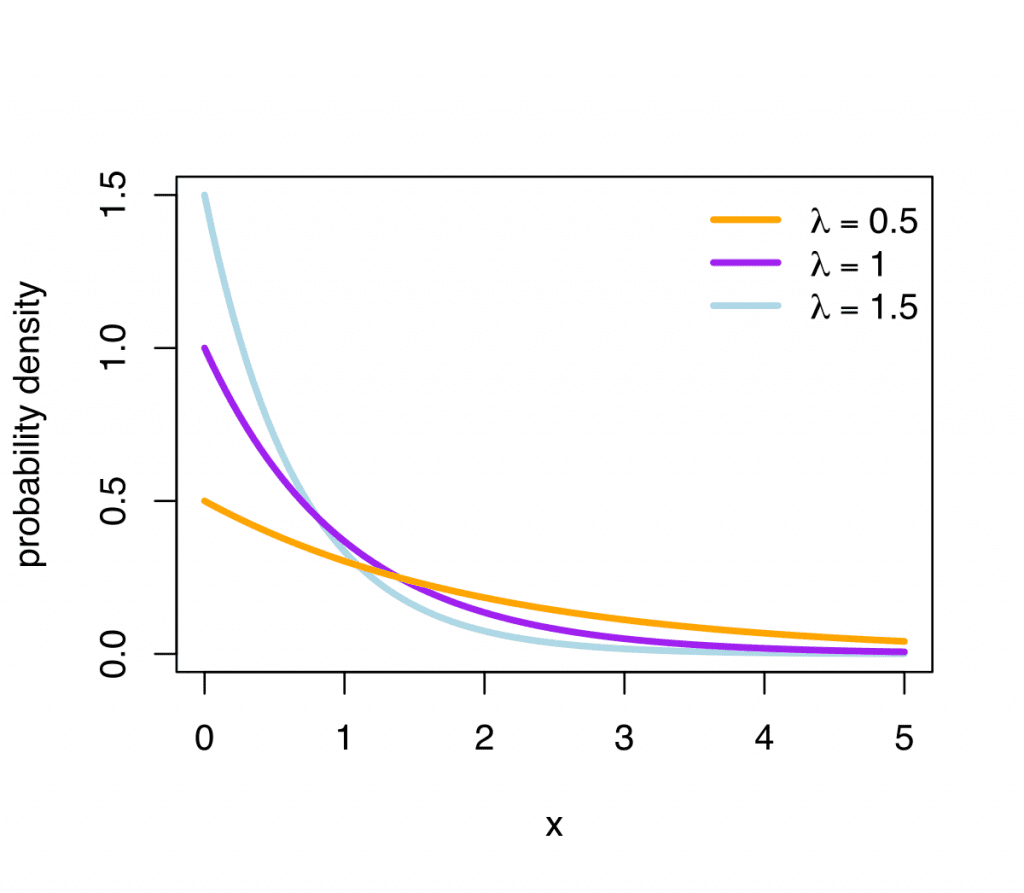This is a very beautiful sample problem from ISI MStat PSB 2012 Problem 3 based on finding the distribution of a random variable . Let's give it a try !!

## Problem- ISI MStat PSB 2012 Problem 3

Let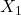and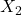be i.i.d. exponential random variables with mean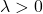.Let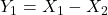andwhere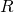is a Bernoulli random variable with parameter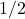and is independent ofand(a) Show that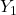and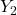have the same distribution.
(b) Obtain the common density function.

### Prerequisites

Cumulative Distribution Function

Bernoulli distribution

Exponential Distribution

## Solution :

Cumulative distribution ofbe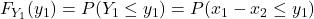,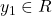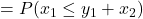Now,Now, ifthen,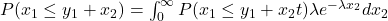=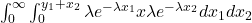=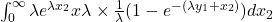==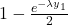Now,then,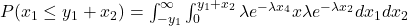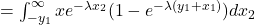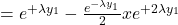Therefore,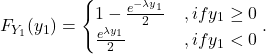Cumulative distribution ofbe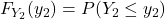,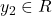=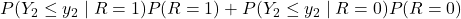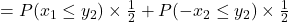==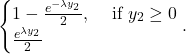since cdf of exponential random Variable, X is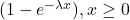Thus bothandhas same distribution
(b)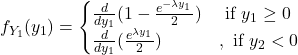=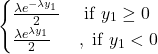Similarly, for.

## Food For Thought

If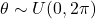then find the distribution of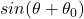, where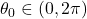.

## Subscribe to Cheenta at Youtube

This is a very beautiful sample problem from ISI MStat PSB 2012 Problem 3 based on finding the distribution of a random variable . Let's give it a try !!

## Problem- ISI MStat PSB 2012 Problem 3

Letandbe i.i.d. exponential random variables with mean.Letandwhereis a Bernoulli random variable with parameterand is independent ofand(a) Show thatandhave the same distribution.
(b) Obtain the common density function.

### Prerequisites

Cumulative Distribution Function

Bernoulli distribution

Exponential Distribution

## Solution :

Cumulative distribution ofbe,Now,Now, ifthen,====Now,then,Therefore,Cumulative distribution ofbe,===since cdf of exponential random Variable, X isThus bothandhas same distribution
(b)=Similarly, for.

## Food For Thought

Ifthen find the distribution of, where.

## Subscribe to Cheenta at Youtube

This site uses Akismet to reduce spam. Learn how your comment data is processed.

### Knowledge Partner